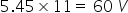Physics
Easy

Question

# Resistors of resistances 10 Ω, 20 Ω, 30 Ω are connected in parallel so that the combination carries a current of 11 A. Potential difference across the combination is

## 60 V30 V120 V6 VHint:

## The correct answer is: 60 V

### When the resistors of resistances 10 Ω, 20 Ω, and 30 Ω are connected in parallel and the combination carries a current of 11 A, the potential difference across the combination is 60V. The potential difference in a parallel combination of resistors is the sum of all the resistances. The resistances of the parallel combination are 10Ω, 20Ω, and 30Ω, and the combination of all can give the potential difference. RT = 5.45 Ω Applying Ohm's law, V= IR,#### With Turito Foundation.#### Get an Expert Advice From Turito.